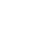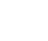# Power BI DAX

Home  >>  Power BI  >>  Power BI DAX

# Power BI DAX14
May,20180

DAX(Data Analysis Expressions)

Calculation Types:

1. Inside the expression always call the table name in front of the column name Table name has to be in single quotes and Column names has to be in Square brackets. Example: ‘Table Name'[Column Name]
2. New Columns: Will give the computed result in Row by Row. Column is required to slice or filter the value. Columns Consume memory
3. New Measures: Written using DAX. Do not work row by row. Instead, use tables and aggregators. Do not have the current row concept. It Aggregates the measure first and does the computation next. Use Measure to Calculate Percentages, Ratios and complex aggregations. Measure consumes CPU. Example: Measure Name = sum(‘sales'[amount]) * sum(‘sales'[margin])

Functions:

The <<X>> Aggregation Function: Using this Aggregation X function we can avoid creating Calculated Columns. Example: SUMX(),COUNTX(),MAXX(),MINX(),AVGX()..ETC

Counting Values: Useful to count values

• COUNT (Only for numeric columns)
• COUNTA (Counts anything but blanks)
• COUNTBLANK (Counts blanks)
• COUNTROWS (rows in a table)
• DISTINCTCOUNT (Performs distinct Count)

Logical Functions:

• AND
• OR
• NOT
• IF
• IFERROR

Information Functions:

• ISBLANK
• ISNUMBER
• ISTEXT
• ISNONTEXT
• ISERROR

DIVIDE Functions:

Divide function useful to avoid using IF inside an expression to check for zero denominators. Example: DIVIDE (‘Sales'[Gross Margin],’Sales'[Sales Amount],0)

Variables:

• Very useful to avoid repeating subexpressions inside the code. Example : Measure := VAR TotalQuatity = SUM (‘Sales[Quantity]’) RETURN IF(TotalQuanlty> 1000,TotalQuantity * 0.95, TotalQuantity * 1.25)

Filter Function:

• Restricts the number of rows of a table
• Returns a table
• Can be iterated by an <<X>> function
• Needs a table as input. The input can be another filter

ALL Function:

1. Returns all the rows of a table
2. Ignore Filter Context
3. Returns a table
4. Can be iterated by an <<X>> function
5. Needs a table as input. Can be used with a single column. Example: ALL (‘Customers'[CustomerName]). The Result contains a table with one column.
6. ALL with many columns. It returns a table with all the values of all the columns passed as parameters.
7. Mixing Filters: SUMX(FILTER(ALL(‘Orders’),’Orders[Channel]=”Internet”),’Orders'[Amount])